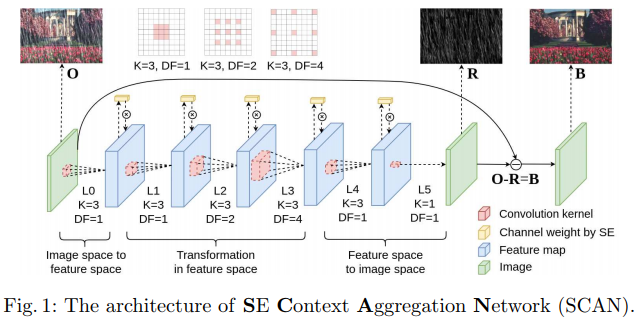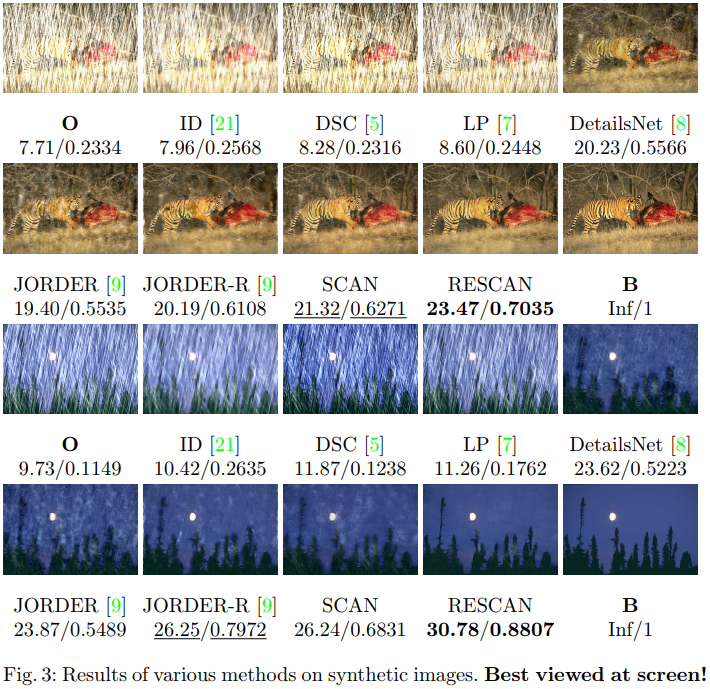The authors propose a convolutional recurrent model for deraining.

Their recurrent cell, similar to GRU, is a ResBlock with Squeeze-and-Excite. Each cell predicts the rain to be removed and the next iteration works on the image minus the rain predicted.Their loss uses the standard squared error between the groundtruth rain streaks and the prediction while also including a coherence loss between predictions at each layer.

$L(O_{S}, \overline{O}) = \sum_{s=1}^{S} \Vert ( \sum_k^s O_{k} - \overline{O}) \Vert ^2$

Where $$O_S$$ are all the outputs at different stages and $$\overline{O}$$ is the groundtruth.

In the end they get a 10% boost on SSIM on Rain100H.Code